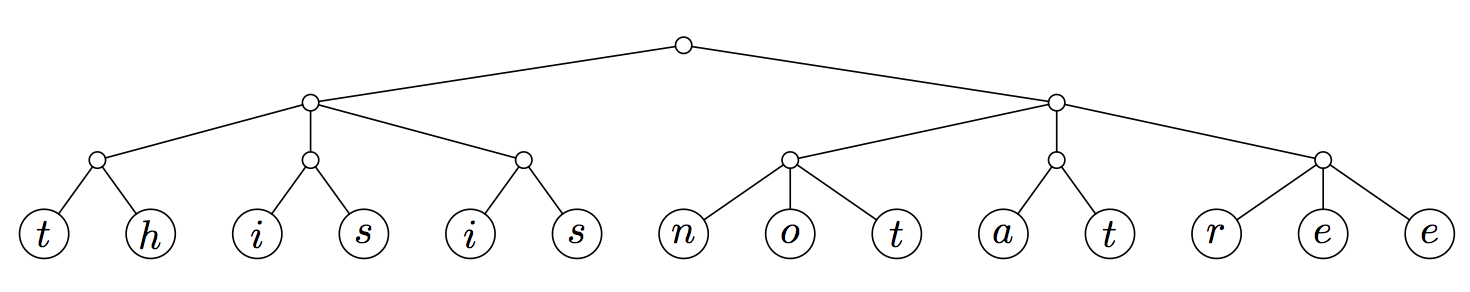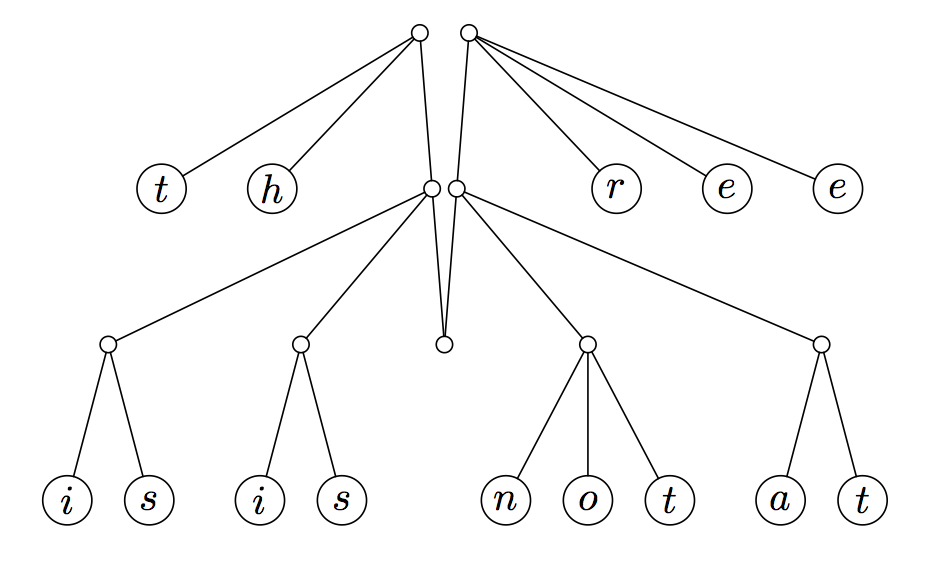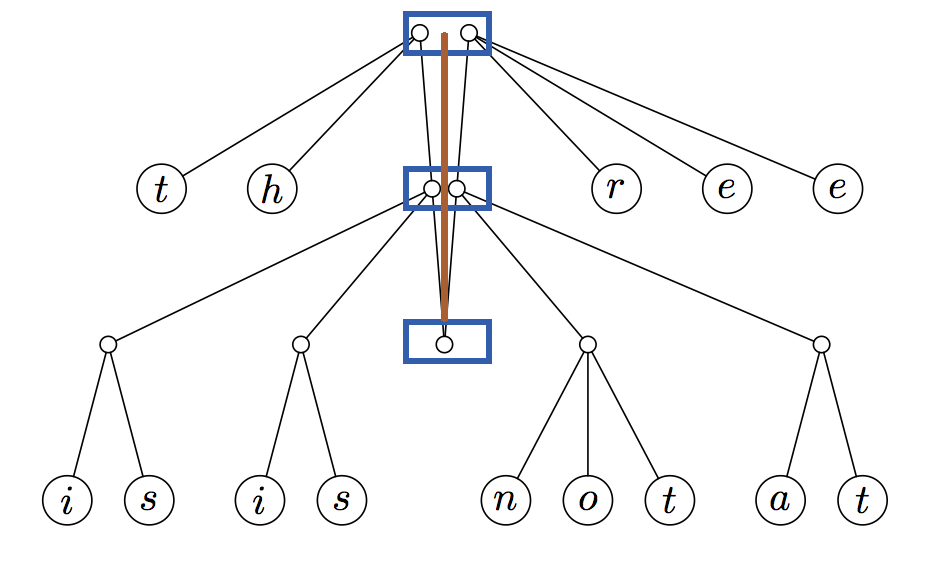# 手指树

## 基本结构

1. 手指树的深度由下到上计算。
2. 手指树的第一级，即树的叶节点，仅包含值，深度为 。第二级为深度 。第三级为深度 ，依此类推。
3. 离根越近，节点指向的原始树（在它是手指树之前的树）的子树越深。这样，沿着树向下工作就是从叶子到树的根，这与典型的树数据结构相反。为了获得这种的结构，我们必须确保原始树具有统一的深度。在声明节点对象时，必须通过子节点的类型进行参数化。深度为 及以上的脊椎上的节点指向树，通过这种参数化，它们可以由嵌套节点表示。

### 将一棵树变成手指树

2-3 树 是一种树状数据结构，其中每个带有子节点（内部节点）的节点具有两个子节点（ 节点）和一个数据元素或三个子节点（ 节点）和两个数据元素。2-3 树是 阶 B 树。树外部的节点（叶节点）没有子节点和一两个数据元素。1 2 3 4 5 6 data FingerTree a = Empty | Single a | Deep (Digit a) (FingerTree (Node a)) (Digit a) data Digit a = One a | Two a a | Three a a a | Four a a a a data Node a = Node2 a a | Node3 a a a 

 1 type Digit a = One a | Two a a | Three a a a | Four a a a a 

### 双向队列操作

1. 用 2-3 个节点替换对提供了足够的灵活性来支持有效的串联。（为了保持恒定时间的双端队列操作，必须将 Digit 扩展为四。）
2. 用幺半群（monoid）注释内部节点允许有效的分裂。
 1 2 3 4 5 data ImplicitDeque a = Empty | Single a | Deep (Digit a) (ImplicitDeque (a, a)) (Digit a) data Digit a = One a | Two a a | Three a a a 

## 时间复杂度

const,snocO(1)O(logn)O(1)/O(n)O(n)
viewl,viewrO(1)O(logn)O(1)/O(n)O(1)
measure/lengthO(1)O(1)O(n)O(1)
appendO(log min(l1, l2))O(logn)O(n)O(m+n)
splitO(log min(n, l-n))O(logn)O(n)O(1)
replicateO(log n)O(logn)O(n)O(n)
fromList,toList,reverseO(l)/O(l)/O(l)O(l)O(1)/O(1)/O(n)O(n)
indexO(log min(n, l-n))O(logn)O(n)O(1)